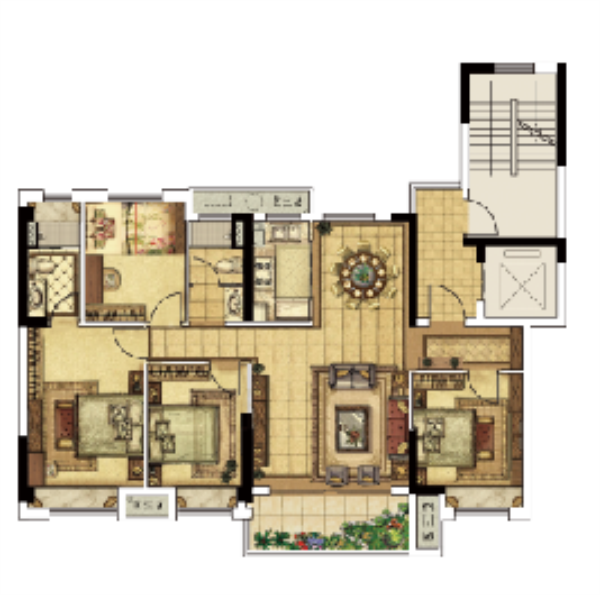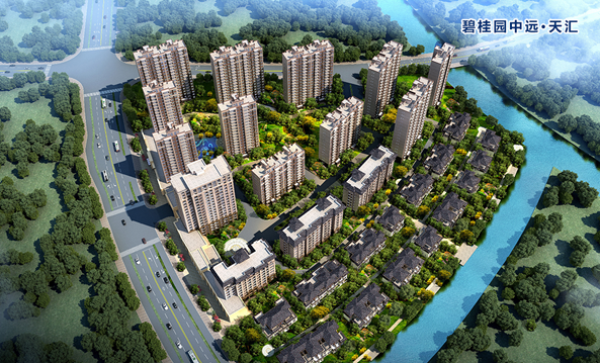## 网站地图## 长兴碧桂园中远•天汇

### 湖州

#### 地址

• 资阳
• 本溪
• 北流
• 万载
• 慈溪
• 长春
• 通化
• 西昌
• 丰城
• 阳春
• 鹤山
• 江油
• 辛集
• 辽源
• 衡水
• 黔西南州
• 黔东南州
• 石家庄
• 唐山
• 秦皇岛
• 邯郸
• 邢台
• 保定
• 张家口
• 承德
• 沧州
• 廊坊
• 黄骅
• 迁安
• 怀来
• 洛阳
• 驻马店
• 郑州
• 开封
• 平顶山
• 安阳
• 鹤壁
• 新乡
• 焦作
• 濮阳
• 许昌
• 漯河
• 三门峡
• 南阳
• 商丘
• 信阳
• 周口
• 武汉
• 黄石
• 十堰
• 宜昌
• 襄阳
• 荆州
• 荆门
• 鄂州
• 仙桃
• 潜江
• 黄冈
• 随州
• 咸宁
• 恩施
• 怀化
• 长沙
• 株洲
• 湘潭
• 湘西州
• 张家界
• 益阳
• 郴州
• 衡阳
• 岳阳
• 常德
• 娄底
• 徐州
• 南京
• 无锡
• 镇江
• 泰州
• 淮安
• 舟山
• 黔西南布依族苗族自治州
• 临沂
• 凉山
• 普洱
• 红河
• 锦州
• 葫芦岛
• 东方
• 咸阳
• 丽水
• 定安县
• 吕梁
• 来宾
• 河池
• 宜宾
• 重庆市
• 雅安
• 潮州
• 大理
• 巴中
• 桂林
• 贵港
• 百色
• 柳州
• 贺州
• 儋州
• 福清
• 威海
• 烟台
• 乐山
• 金华
• 永泰
• 潍坊
• 滨州
• 德州
• 临汾
• 日照
• 眉山
• 武威
• 乌鲁木齐
• 保山
• 临沧
• 防城港
• 达州
• 遂宁
• 绵阳
• 淮南
• 宝鸡
• 枣庄
• 桂平
• 景德镇
• 临夏
• 聊城
• 崇左
• 马鞍山
• 安顺
• 北海
• 菏泽
• 铜陵
• 黔南州
• 蚌埠
• 嘉兴
• 淮北
• 都匀
• 南宁
• 兴义
• 南平
• 松溪
• 吉安
• 大同
• 陆丰
• 厦门
• 铜仁
• 银川
• 昭通
• 包头
• 遵化
• 漳州
• 深圳
• 霸州
• 香港
• 合肥
• 抚州
• 琼海
• 定西
• 南昌
• 宿州
• 文昌
• 临高县
• 陵水
• 海口
• 博鳌
• 三亚
• 绥化
• 哈尔滨
• 杭州
• 绍兴
• 宁波
• 温州
• 衢州
• 湖州
• 台州
• 天津
• 沈阳
• 大连
• 通辽
• 兴安盟
• 呼伦贝尔
• 运城
• 晋中
• 太原
• 南通
• 宿迁
• 扬州
• 盐城
• 连云港
• 苏州
• 常州
• 安庆
• 黄山
• 巢湖
• 芜湖
• 池州
• 宣城
• 六安
• 古田
• 无为
• 桐城
• 亳州
• 南陵
• 庐江
• 阜阳
• 滁州
• 孝感
• 天门
• 邵阳
• 永州
• 平果
• 玉林
• 梧州
• 钦州
• 梅州
• 河源
• 云浮
• 东莞
• 汕头
• 中山
• 清远
• 惠州
• 韶关
• 肇庆
• 阳江
• 汕尾
• 茂名
• 江门
• 佛山
• 广州
• 雷州
• 英德
• 湛江
• 揭阳
• 珠海
• 信宜
• 济南
• 淄博
• 青岛
• 泰安
• 济宁
• 马来西亚雪兰莪州
• 马来西亚柔佛州
• 永春
• 南安
• 三明
• 宁德
• 武夷山
• 福鼎
• 莆田
• 建瓯
• 漳浦
• 福安
• 福州
• 泉州
• 龙岩
• 兰州
• 张掖
• 天水
• 白银
• 贵阳
• 仁怀
• 毕节
• 凯里
• 六盘水
• 遵义
• 广元
• 德阳
• 南充
• 泸州
• 攀枝花
• 广安
• 成都
• 宜春
• 萍乡
• 九江
• 赣州
• 上饶
• 曲靖
• 楚雄
• 昆明
• 白城
• 北京
• 澳洲莱德
• 西安
• 渭南
• 上海
• 西宁

核心优势：

配套

社区：儿童乐园、E邮柜、智能门锁、直饮水系统、电梯入户、智能车辆识别;

周边：举目繁华一站式生活服务

项目周边商圈林立，紧邻18万方海洋城购物中心，距离浙北商业广场1.4KM，利时广场2.0KM，畅享一站式生活服务。

2公里范围内有实验初中、长兴县政府、长兴太湖高级中学、南方大厦等，周边3.5KM交通圈涵盖了餐饮、购物、休闲、娱乐、文化、教育等场所，生活便利。

更有规划30万方的雨润商业综合体并且在项目的北侧外部政府意向引进一所12年制的高级学校，区位繁华指日可待。

环境

醉美园林

园林领军大师梁国坤设计团队，甄选石材树种因地而建，利用自然风光、丰富山体资源，步步怡景，打造真正多重立体园林景观。

以木、大乔、小乔、灌、花、草等多重植物，形成纷叠错落的立体景致，全冠移植多种树木至园中，如银杏、水杉、杨梅等。项目的园林重点在于“一步一景”，打造一个“世外桃源”，蜿蜒绿道、缤纷花床、水边休憩茶座互为景中景，如诗如画梦境，在满足您欣赏美景的同时，也可以很好的过滤城市噪音和灰尘。

其他：

清新水系、秀丽公园环保，使居住更亲近自然;智慧至境云宅;多开间朝南；毗邻高铁站、杭宁高速，连接长三角经济脉络。

户型：YJ115

三开间朝南

南向大尺度开间，阔绰视野，点亮生活

一梯一户，尽享尊贵

南北通透

客厅南北通透，通风采光性佳

南北双阳台

北向独立家政阳台

南向独立大面宽景观阳台YJ140

三开间朝南

南向大尺度开间，阔绰视野，点亮生活

一梯一户，尽享尊贵

南北通透

客厅南北通透，通风采光性佳

多飘窗设计

卧室均有大飘窗

延展起居场所，提高空间利用率

鸟瞰图+区位图+透视图: